• 本期视频时长约70分钟，通过具体的系统分析案例，详细地讲解了连续系统的三种时域分析方法：系统微分方程求解法，基于传递函数的lsim求解方法，基于冲激响应的卷积积分求解法。视频的最后，还对各种方法进行了总结和...
• 实 验 1- 连 续 时 间 系 统 的 时 域 分 析 及 ma t l a b 实 现 精品文档 实验一 连续时间系统的时域分析matlab 实现 一实验目的 熟悉连续时间系统零状态响应的求解及 matlab 实现用卷积方法求零状态 响应 , ...
• 卷积积分是线性系统时域分析最基本方法，为加深对线性系统时域分析的理解，下面先讨论卷积积分 所用matlab函数： stepfun(t,t0) 阶跃函数，t0为起点为1； conv(f1,f2) 卷积； ezplot(dslove(‘微分方程’，‘零...
前言
卷积积分是线性系统时域分析最基本的方法，为加深对线性系统时域分析的理解，下面先讨论卷积积分
所用matlab函数：
stepfun(t,t0) 阶跃函数，t0为起点为1；
conv(f1,f2) 卷积；
ezplot(dslove(‘微分方程’，‘零状态’),[A,B])函数ezplot无需数据准备，可以直接画出函数的图形;ezplot(f,[A,B]):f是关于x的函数(A<x<B) 且自动将函数表达式当做标题显示
sys=tf(b,a);  传输函数，分子的系数为b，分母的系数为a;
impulse(sys,t)  求其冲激响应;
step(sys,t)  求其阶跃响应;
y=lsim(sys,f,t,zi);   对系统输出进行仿真求其输入f的全响应，zi为初始条件 ；
1.分子分母形式：lsim(num,den,u,t)；
2.传递函数形式：lsim(sys,u,t)  ；
3.状态空间形式： [A B C D]=tf2ss(b,a); %将传递函数转换到状态空间表达式lsim(A,B,C,D,u,t) %然后求其响应
或者将状态空间用sys表示：sys=ss(A,B,C,D);lsim(sys,u,t)
不加zi初始条件，则是零状态响应；
卷积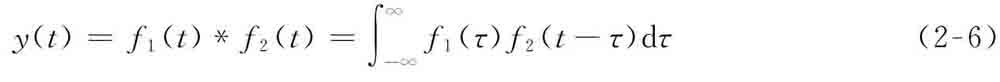这是卷积的表达式，
1.任意函数与冲激函数卷积等于函数本身
2.任意函数与冲激函数延迟t0卷积等于函数延迟t0
3.任意函数与阶跃函数卷积等于函数的积分
卷积的性质
1.交换律 2.分配率 3.结合律 4.时移性质 5.微积分性质
常用信号的卷积公式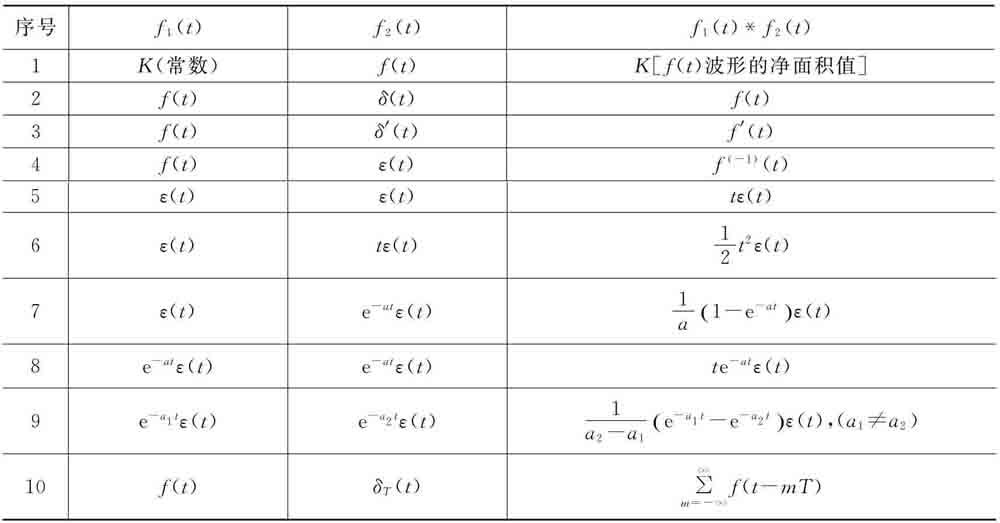例题来深入理解卷积
NO.1
用MATLAB画出门函数3[ε（t+1）–ε（t–2）]与指数函数2e–2t卷积的图形。
解：MATLAB程序如下：
%求f1和f2的卷积
T=0.01;
t1=-2:T:2;
t2=0:T:3;   %生成两个待卷积信号的时间向量
f1=3*(stepfun(t1,-1)-stepfun(t1,2));
f2=2*exp(-2*t2);
y=conv(f1,f2);
y=y*T;  **%卷积运算求的是重叠区域的值相乘求积分，即是面积，所以要乘上时间间隔**
t=-2:T:5;    **%序列y的非零值的宽度 m+n-1   t11+t21:t12:t22**
subplot(311)
plot(t1,f1);
axis([-2 5 min(f1) max(f1)+0.5]);
title('f1')
subplot(312)
plot(t2,f2);
axis([-2 5 min(f2) max(f2)+0.5]);
title('f2')
line([0,0],[0,max(f2)]);
subplot(313)
plot(t,y);
axis([-2 5 min(y) max(y)+0.5]);
title('卷积结果y')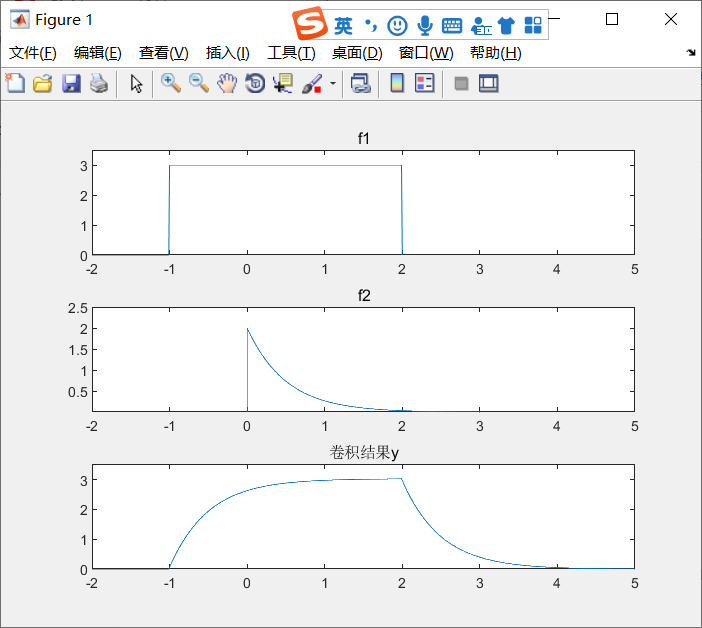NO.2
画出门函数[ε（t+1）–ε（t–2）]与门函数3[ε（t）–ε（t–2）]卷积的图形。
解：MATLAB程序如下：
%求f1和f2的卷积
T=0.01;
t1=-2:T:2;
t2=0:T:3;   %生成两个待卷积信号的时间向量
f1=(stepfun(t1,-1)-stepfun(t1,2));
f2=3*(stepfun(t2,0)-stepfun(t2,2));
y=conv(f1,f2);
y=y*T;  %卷积运算求的是重叠区域的值相乘求积分，即是面积，所以要乘上时间间隔
t=-2:T:5;    %序列y的非零值的宽度 m+n-1   t11+t21:t12:t22
subplot(311)
plot(t1,f1);
axis([-2 5 min(f1) max(f1)+0.5]);
title('f1')
subplot(312)
plot(t2,f2);
axis([-2 5 min(f2) max(f2)+0.5]);
title('f2')
line([0,0],[0,max(f2)]);
subplot(313)
plot(t,y);
axis([-2 5 min(y) max(y)+0.5]);
title('卷积结果y')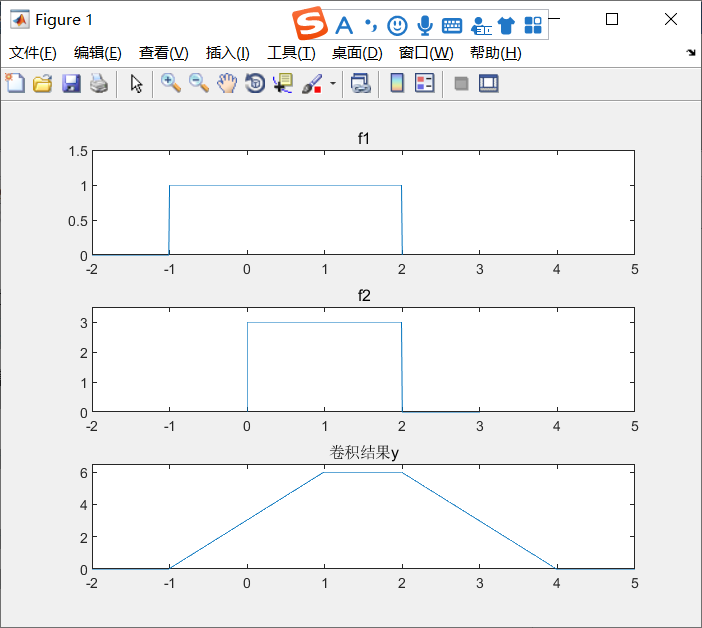NO.3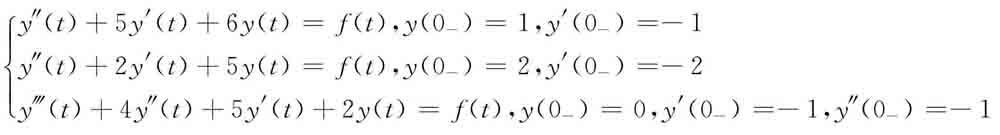求系统零输入响应
%求解系统的零输入响应
subplot(311);
ezplot(dsolve('D2y+5*Dy+6*y=0','y(0)=1,Dy(0)=-1'),[0 8])    %函数ezplot无需数据准备，可以直接画出函数的图形，画隐函数图形很方便
% ezplot(f,[A,B]):f是关于x的函数(A<x<B) 且自动将函数表达式当做标题显示
axis auto
subplot(312);
ezplot(dsolve('D2y+2*Dy+5*y=0','y(0)=2,Dy(0)=-2'),[0 8])
axis auto
subplot(313);
ezplot(dsolve('D3y+4*D2y+5*Dy+2*y=0','y(0)=0,Dy(0)=-1,D2y(0)=-1'),[0 8])
axis auto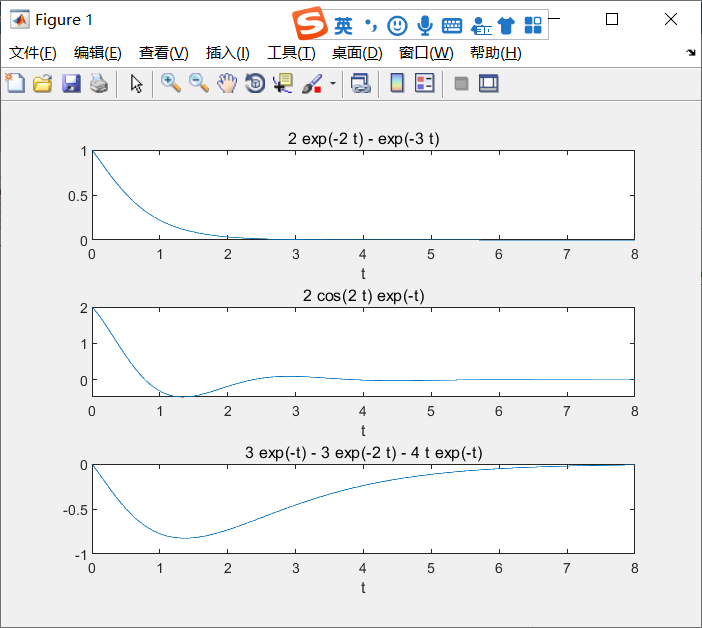NO.4
已知系统为i’’(t)+5i’(t)+6i(t)=e’(t),利用MATLAB求系统的冲激响应和阶跃响应。
解：求系统零状态响应的MATLAB程序如下：
%求解系统的冲激响应和阶跃响应
%D2i+5*Di+6*i=De
b=[1 0];
a=[1 5 6];
sys=tf(b,a);  %传输函数，分子的系数为b，分母的系数为a
t=0:0.1:10;
y=impulse(sys,t) %求其冲激响应
plot(t,y)
figure
y=step(sys,t) %求其阶跃响应
plot(t,y)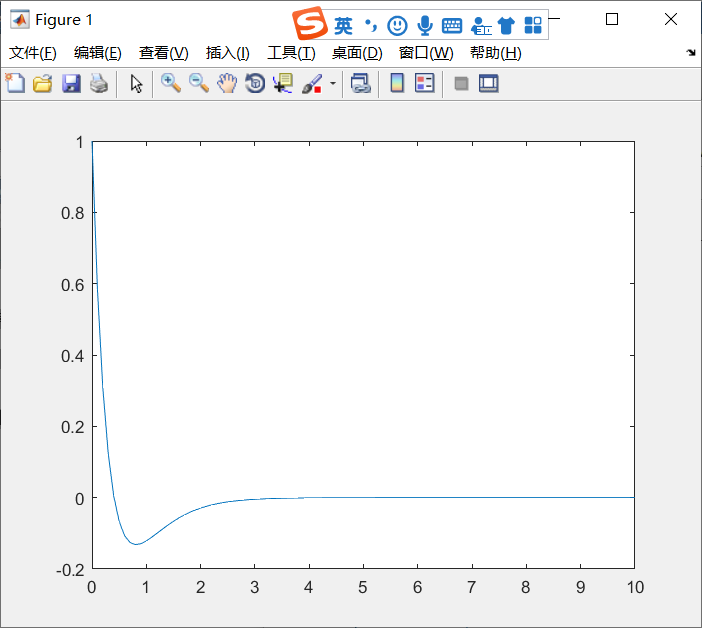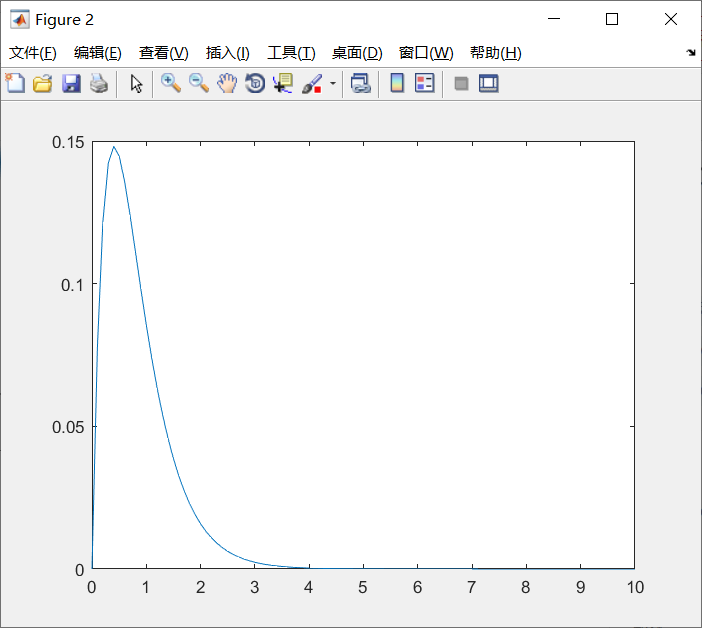NO.5
利用MATLAB解方程y″（t）+4y′（t）+3y（t）=f′（t）+3f（t），f（t）=e–tε（t）的零状态响应。
解：求系统零状态响应的MATLAB程序为：
%求解y''（t）+4y'(t)+3y(t)=f'(t)+3f(t),f(t)=exp(-t)u(t) 零状态响应
clear all;
ts=0;te=10;dt=0.01;
sys=tf([1 3],[1 4 3]);
t=ts:dt:te;
x=exp(-1*t);
y=lsim(sys,x,t)                 %计算零状态响应?x为输入 t为时间向量
plot(t,y);
xlabel('t(sec)');ylabel('y(t)');
axis([t(1) t(length(t)) -0.5 0.5]);
grid on;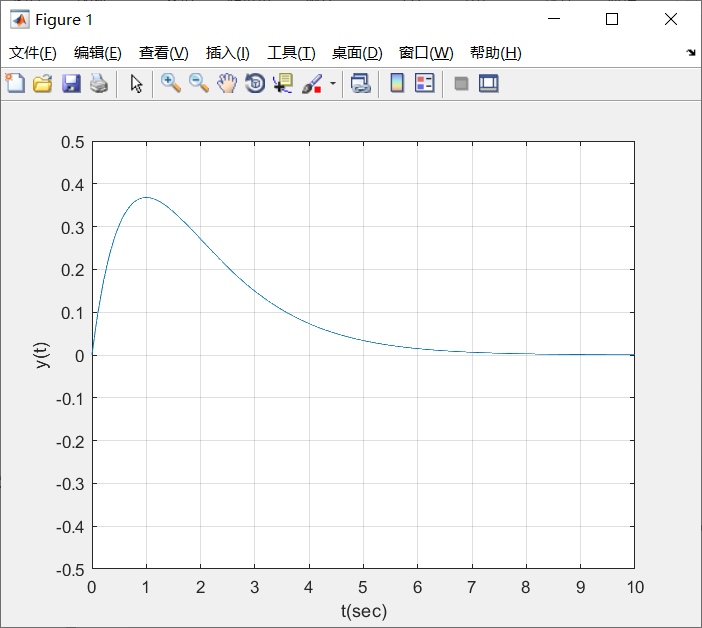NO.6
已知系统为y″（t）+y（t）=f（t），初始条件y′（0）=–1，y（0）=0，激励f（t）=cos2πt，利用MATLAB求系统的完全响应。
%求y''(t)+y(t)=f(t) y'(0)=-1 y(0)=0 f(t)=cos(2pit) 完全响应
clear;
b=;a=[1 0 1];       %b是分子的系数矩阵,a是分母的系数矩阵??
sys=tf(b,a);
t=0:0.1:30;
f=cos(t);
zi=[-1 0];   %初始条件
y=lsim(sys,f,t,zi);  %1.分子分母形式lsim(num,den,u,t)
%2.传递函数形式 lsim(sys,u,t)  3.状态空间形式lsim(A,B,C,D,u,t)  [A B C D]=tf2ss(b,a); sys=ss(A,B,C,D);  不加初始条件就是零状态响应
plot(t,y);
xlabel('时间(t)');
ylabel('y(t)');   title('系统的全响应');   line([0,30],[0,0]);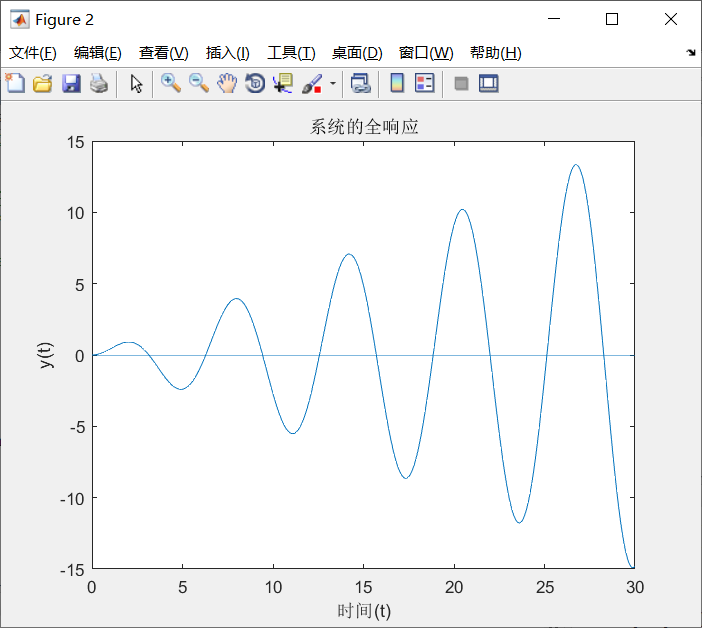展开全文• 1.符号求解求解微分方程，信号零输入与零状态： 实验代码如下： 2.符号求解求解微分方程，信号零输入与零状态以及完全响应： eq= 'D2y+3*Dy+2*y=0'; cond= 'y(0)=0,Dy(0)=2'; yzi=dsolve(eq,cond);yzi=...
1.符号求解求解微分方程，信号的零输入与零状态：
实验代码如下：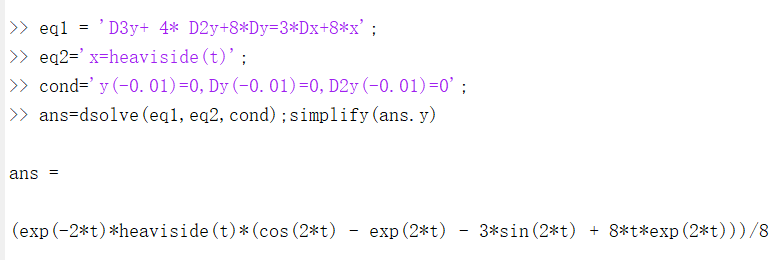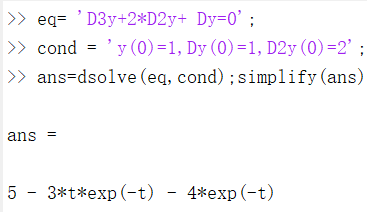2.符号求解求解微分方程，信号的零输入与零状态以及完全响应：

eq= 'D2y+3*Dy+2*y=0';
cond= 'y(0)=0,Dy(0)=2';
yzi=dsolve(eq,cond);yzi=simplify(yzi);
eq1='D2y+3*Dy+2*y=Dx+3*x';
eq2='x=exp(-3*t)*heaviside(t)';
cond1='y(-0.001)=0,Dy(-0.001)=0';
yzs=dsolve(eq1,eq2,cond1);yzs=simplify(yzs.y);
yt=simplify(yzi+yzs);
subplot(221),ezplot(yzi,[0,8]),grid on,title('零输入响应');
subplot(222),ezplot(yzs,[0,8]),grid on,title('零状态响应');
subplot(223),ezplot(yt,[0,8]),grid on,title('完全响应');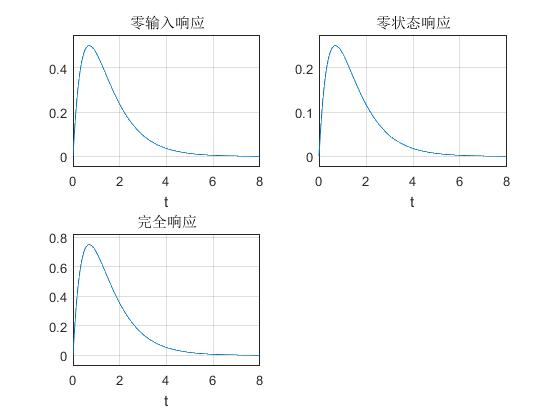3.连续时间系统零状态响应的数值求解1：

ts=0;te=5;dt=0.01;
sys=tf(,[1,5,6]);
t=ts:dt:te;
>> f=10*sin(2*pi*t).*(t>=0);
>> y=lsim(sys,f,t);
>> plot(t,y),grid on
>> xlabel('Times(sec)'),ylabel('y(t)'),title('零状态响应')

绘制图像如下：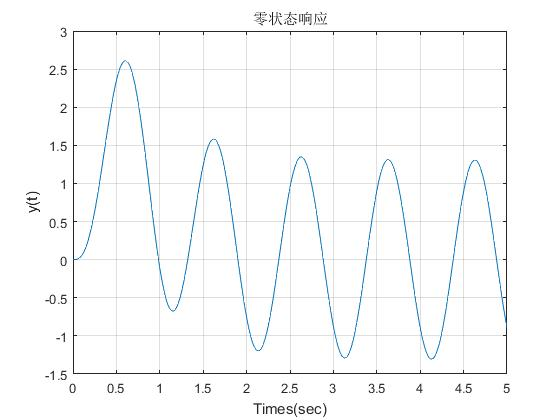4.连续时间系统零状态响应的数值求解2：

ts=0;te=8;dt=0.01;
>> sys=tf([1,3],[1,3,2]);
>> t=ts:dt:te;
>> f=exp(-3*t).*(t>=0);
>> y=lsim(sys,f,t);
>> plot(t,y);
>> grid on
>> axis([0 8 -0.02 0.27]);
>> xlabel('Times(sec)'),ylabel('y(t)');
>> title('零状态响应')

实验结果如下图：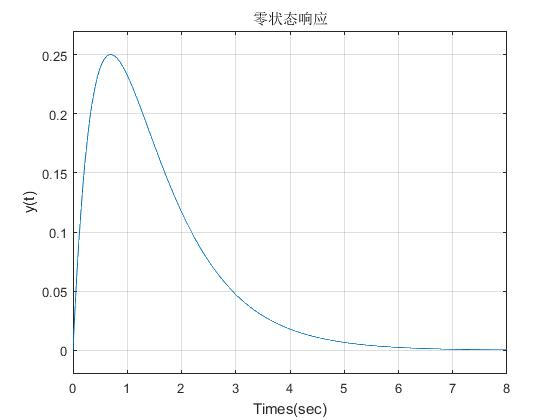5.
连续时间系统冲激响应与阶跃响应的求解：

t=0:0.01:4;
sys=tf([1,16],[1,2,32]);
h=impulse(sys,t);%冲激响应
g=step(sys,t);%阶跃响应
>> subplot(221),plot(t,h),grid on,xlabel('Time(sec)'),ylabel('h(t)'),title('冲激响应');
>> subplot(222),plot(t,g),grid on,xlabel('Time(sec)'),ylabel('g(t)'),title('阶跃响应');


实验图：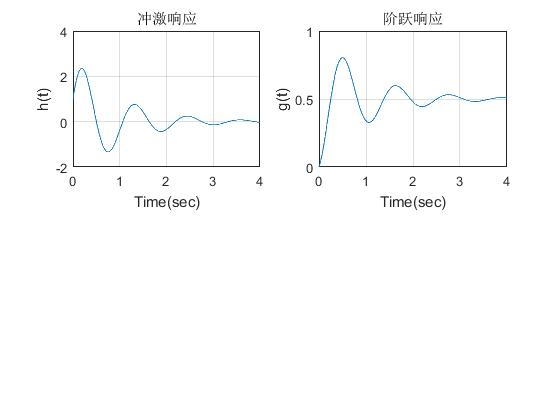展开全文• 1、dsolve函数 在MATLAB中求解常系数微分方程可以利用dsolve(‘eq1,eq2,…’,’cond1,cond2,…’,’v’)函数，其中参数eq表示各个微分方程，微分和...利用dslve()函数可以求出系统微分方程零输入性响应和零状态响...
1、零状态响应
题目如下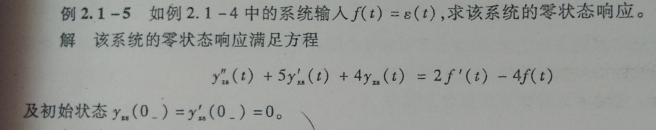我们用lsim(sys,f,t)来求解零状态响应，关于此函数用法看下图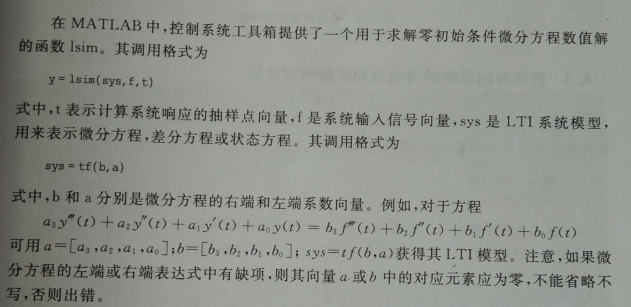代码如下

ts=0;te=5;dt=0.01;
sys=tf([2,-4],[1 5 4]);   %得到LTI系统模型，其实就是所要求解的微分方程的各项系数
t=ts:dt:te;
f=heaviside(t);           %设定激励为阶跃信号
yzs=lsim(sys,f,t);        %求解零状态响应
plot(t,yzs)
grid on
xlabel('t');
ylabel('yzs(t)');
title('零状态响应曲线')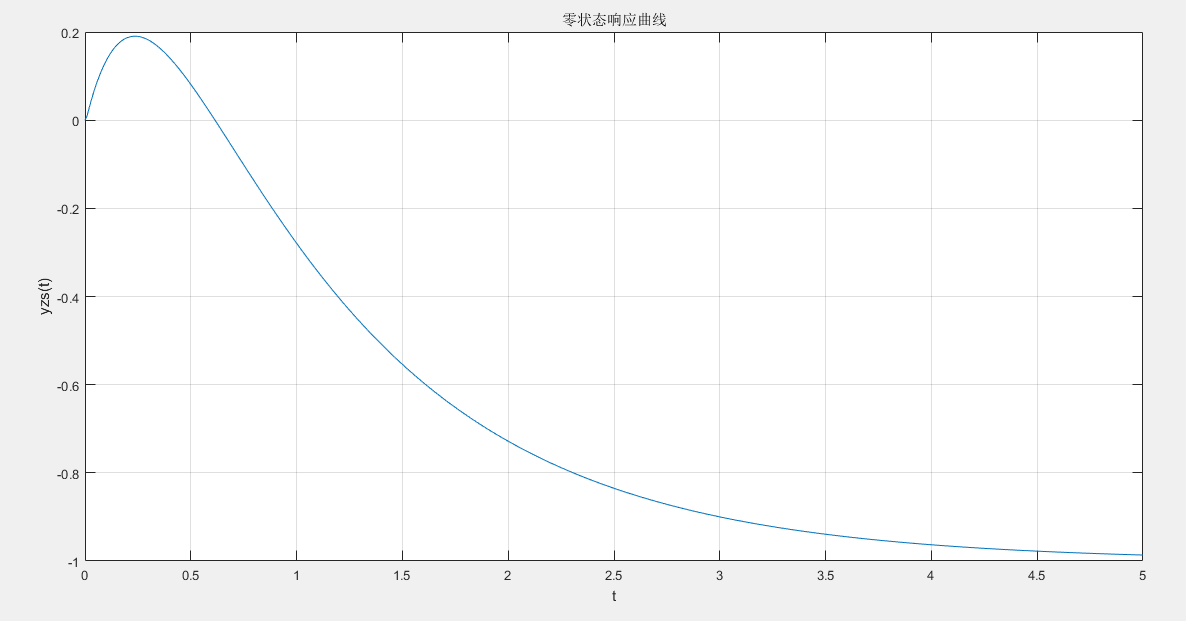2、零输入响应
题目如下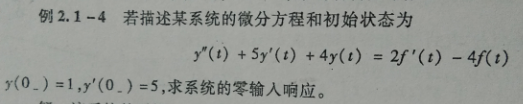我们用dsolve(‘eq1,eq2,…’,’cond1,cond2,…’,’v’)函数来求解，关于此函数用法看下图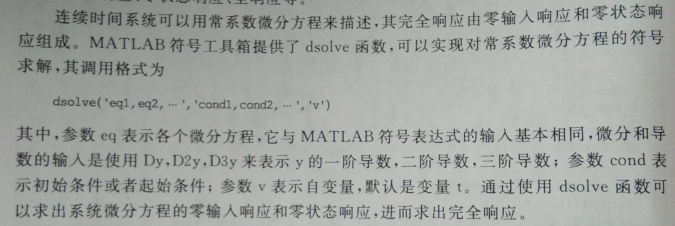代码如下

eq='D2y+5*Dy+4*y=0';      %求解零输入响应时，激励应该令其为0
cond='y(0)=1,Dy(0)=5';    %初始条件
yzi=dsolve(eq,cond)

结果如下

yzi =

3*exp(-t) - 2*exp(-4*t)

3、冲激响应和阶跃响应
如果激励是冲激函数或者阶跃函数，我们除了用上面提到的两个函数（lsim(),dsolve()）外，还可以利用专门求解冲激响应和阶跃响应的函数，冲激响应h=impulse(sys,t)，阶跃响应g=step(sys,t)
代码如下

t=0:0.002:4;
sys=tf([1,32],[1,4,64]);      %LTI系统
h=impulse(sys,t);             %求解冲激响应
g=step(sys,t);                %求解阶跃响应
subplot(211)
plot(t,h)
grid on
xlabel('时间/s')
ylabel('h(t)');
title('冲激响应');
subplot(212)
plot(t,g)
grid on
xlabel('时间/s')
ylabel('g(t)');
title('阶跃响应')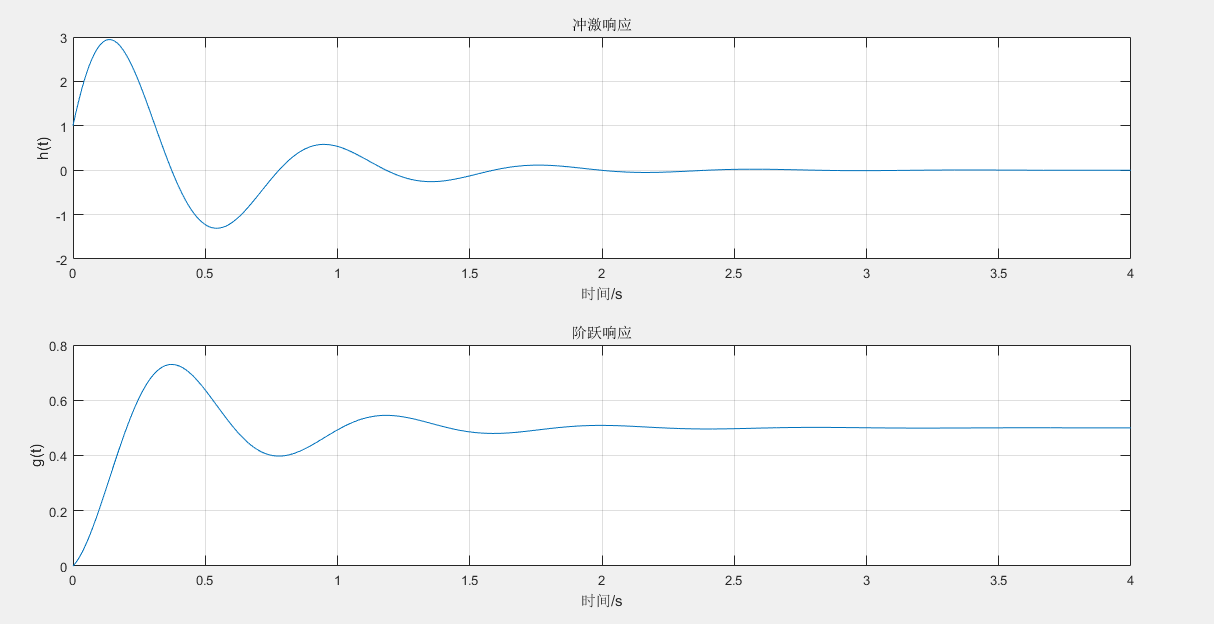4、卷积
信号的卷积运算有符号算法和数值算法，此处采用数值计算法，需要调用MATLAB中的conv()函数来近似计算信号的卷积积分
例：用数值计算法求f1(t)=u(t)-0.5u(t-2)与f2(t)=2e^(-3t)u(t)的卷积积分，其中u(t)代表阶跃信号
代码如下

dt=0.01;
t=-1:dt:2.5;
f1=heaviside(t)-0.5*heaviside(t-2);
f2=2*exp(-3*t).*heaviside(t);
f=conv(f1,f2)*dt;
n=length(f);
tt=(0:n-1)*dt-2;
subplot(221)
plot(t,f1);
grid on
axis([-1,2.5,-0.2,1.2])
title('f1(t)');
xlabel('t')
ylabel('f1(t)');
subplot(222)
plot(t,f2);
grid on
axis([-1,2.5,-0.2,1.2])
title('f2(t)');
xlabel('t')
ylabel('f2(t)');
subplot(212)
plot(tt,f)
grid on
title('卷积积分')
xlabel('t')
ylabel('f3(t)')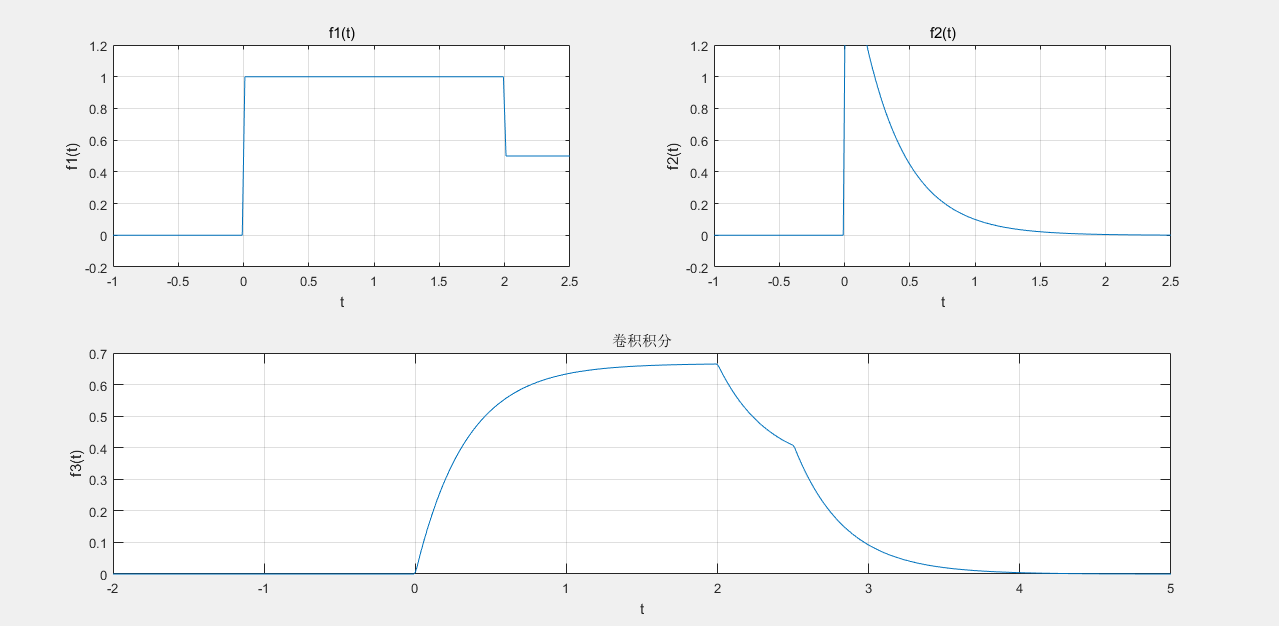展开全文• 上机实验 2 连续 LTI系统的时域分析 一实验目的 熟悉 LTI 系统在典型激励信号的响应及其特性 熟悉连续 LTI 系统单位冲激响应的求解方法 重点掌握用卷积计算连续时间系统的零状态响应 熟悉 MATLAB相关函数的调用格式...
• 范文 MATLAB课程设计任务书 姓名王* 学号2010*010 题目: 连续时间信号和系统时域分析MATLAB实现 初始条件 MATLAB 7.5.0 Windows XP系统 实验任务 一用MATLAB实现常用连续时间信号的时域波形通过改变参数分析其...
• 1.符号法与数值求解微分方程零输入与零状态响应： %微分方程符号法求解零输入响应 eq='D2y+4*Dy+4*y=0'; cond='Dy(0)=1,y(0)=2'; yzi=dsolve(eq,cond);simplify(yzi) %微分方程符号法求解零状态响应 eq1='D2y+4...
1.符号法与数值求解微分方程的零输入与零状态响应：

%微分方程符号法求解零输入响应
eq='D2y+4*Dy+4*y=0';
cond='Dy(0)=1,y(0)=2';
yzi=dsolve(eq,cond);simplify(yzi)
%微分方程符号法求解零状态响应
eq1='D2y+4*Dy+4*y=Dx+3*x';
eq2='x=exp(-t)*heaviside(t)';
cond='Dy(-0.01)=0,y(-0.01)=0';
yzs=dsolve(eq1,eq2,cond);simplify(yzs.y)
yzi =exp(-2*t)*(5*t + 2)
yzs =(exp(-2*t)*(sign(t) + 1)*(t + 2*exp(t) - 1))/2
%数值求解微分方程的零状态响应
t=0:0.01:4;
>> f=exp(-t).*(t>=0);
>> y=lsim(sys,f,t);
>plot(t,y),grid on

实验结果：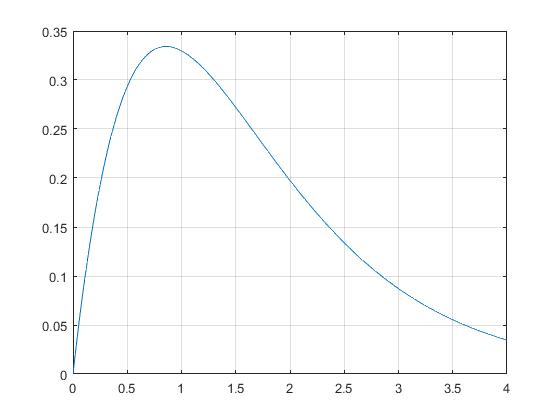2.使用MATLAB命令画出系统的冲激响应与阶跃响应：

clear all
t=0:0.01:8;
sys=tf([1,0],[1,2,2]);
h=impulse(sys,t);
g=step(sys,t);
%绘制冲激响应图像
subplot(121),plot(t,h),grid on
xlabel('Time(sec)'),ylabel('h(t)'),title('冲激响应');
%绘制阶跃响应图像
>> subplot(122),plot(t,g),grid on
>> xlabel('Time(sec)'),ylabel('g(t)'),title('阶跃响应');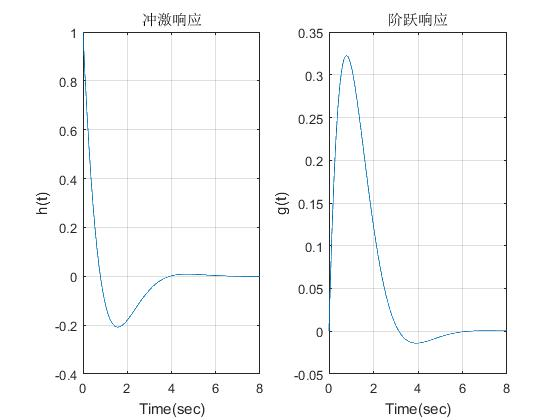展开全文• 本节书摘来自异步社区《MATLAB信号处理超级...2.4 连续时间系统的时域分析 MATLAB信号处理超级学习手册2.4.1 连续时间系统求解连续时间线性非时变系统（LTI）可以用如下的线性常系数微分方程来描述： 系统的响应一般...
• 本人自己编写，小试身手一下，如果有错误还请高手指教
• 课程设计任务书 学生姓名: 专业班级: 指导教师: 工作单位: 题 目: 连续时间信号和系统时域分析MATLAB 实现 初始条件 MATLAB 6.5 要求完成的主要任务 一用 MATLAB 实现常用连续时间信号的时域波形通过改变参数 ...
• 课程设计任务书 学生姓名 : 专业班级 : 指导教师 : 工作单位 : 题 目: 连续时间信号和系统时域分析MATLAB 实现 初始条件 MATLAB 6.5 要求完成的主要任务 一用 MATLAB 实现常用连续时间信号的时域波形通过改变参数...
• 实用标准文案 MATLAB课程设计任务书 姓名王 * 学号 2010*010 题目 : 连续时间信号和系统时域分析MATLAB实现 初始条件 MATLAB 7.5.0 Windows XP系统 实验任务 一用 MATLAB实现常用连续时间信号的时域波形通过改变...# matlab连续时间系统的时域分析matlab 订阅